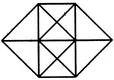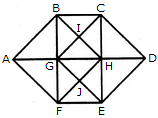# Non Verbal Reasoning - Analytical Reasoning - Discussion

### Discussion :: Analytical Reasoning - Section 1 (Q.No.23)

23.

Find the number of triangles in the given figure.[A]. 20 [B]. 24 [C]. 28 [D]. 32

Explanation:

The figure may be labelled as shown.The simplest triangles are ABG, BIG, BIC, CIH, GIH, CDH, HED, GHJ, HJE, FEJ, GFJ and AGF i.e. 12 in number.

The triangles composed of two components each are ABF, CDE, GBC, BCH, GHG, BHG, GHF, GHE, HEF and GEF i.e. 10 in number.

The triangles composed of three components each are ABH, AFH, CDG and GDE i.e. 4 in number.

The triangles composed of four components each are BHF and CGE i.e. 2 in number.

Total number of triangles in the figure = 12 + 10 + 4 + 2 = 28.

 Shuvam said: (May 4, 2021) How is GHG a triangle? please explain.